# CSET Math Prep

Discussion in 'Single Subject Tests' started by innovationguy, Jan 6, 2019.

Tags:

Joined:
Jul 24, 2004
Messages:
486
2

Jan 6, 2019

Having coached, mentored and tutored hundreds -- if not thousandsof candidates in the last decade and half, Im happy to provide DAILY practice for the CSET. To be clear, these Qs reflect the difficulty of those on the test but the style may (deliberately) differ; the content = subject-matter knowledge you need to succeed is absolutely FAIR GAME.

1. SUBTEST 1: For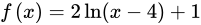, which of the following statements are FALSE? [There may be more than 1 answer. For better comprehension, CORRECT the statements that are incorrect.]
a) The Increasing Interval is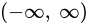.

b) The Domain is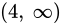.

c) The V.A. is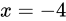d) The H.A. is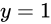.

2. SUBTEST 2. Given the function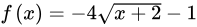, write the equation of a new function,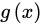AFTER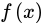is translated 3 units LEFT and 4 units UP.

Answers shall be posted the FOLLOWING dayJay.
https://csetmathguru.weebly.com/

Last edited: Jan 18, 2019
2.3. ### ArtificialScrewRookie

Joined:
Mar 24, 2017
Messages:
15
0

Jan 13, 2019

Off top of my head,
1. C is false, x = 4 is the VA. Logs aren't even defined for less than 0. B is true. D is false, natural log functions do not converge. A is false, the increasing interval is the domain itself.

2. -4 sqrt(x - 1) + 3

Passed these stupid tests a while back.

Joined:
Jul 24, 2004
Messages:
486
2

Jan 15, 2019

Solution to #1.
A is False the log graph does not increase over all Real numbers.
C is False since the V.A. is x = 4.
D is False since the log graph does not have H.A.

Solution #2. is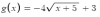since the original function was shifted 2 units LEFT from the parent function, so 3 units FURTHER left would make x = -5 the relevant ordinate.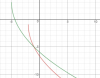Last edited: Feb 19, 2019

Joined:
Jul 24, 2004
Messages:
486
2

Jan 15, 2019

#3. SUBTEST 1. Ifa root of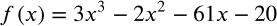, which of the following are also roots of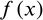? Choose ALL that apply.
1.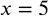2.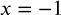3.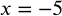4.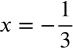5.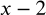6.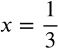#4. SUBTEST 1. The graph of a polynomial function is given below: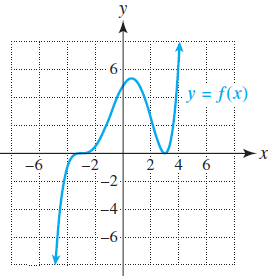Which of the following could be the polynomial function (of lowest degree)?

1.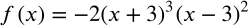2.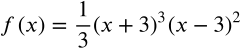3.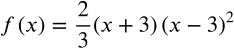4.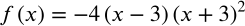Last edited: Feb 18, 2019

Joined:
Jul 24, 2004
Messages:
486
2

Jan 18, 2019

Joined:
Jul 24, 2004
Messages:
486
2

Jan 18, 2019

Last edited: Jan 18, 2019

Joined:
Jul 24, 2004
Messages:
486
2

Jan 18, 2019

#7. SUBTEST 2. A soft drink dispenser can be adjusted to deliver any fixed number of ounces of soft drink. If the machine is operating with a standard deviation in delivery equal to 0.3 oz, what should the mean setting be so that a 12 oz cup will overflow less than 1% of the time?
1. 11.23 oz
2. 11.3 oz
3. 11.7 oz.
4. 12.7 oz
5. 12.77 oz

Joined:
Jul 24, 2004
Messages:
486
2

Jan 18, 2019

Solution to #3. Since x = -4 is a root, then x + 4 is a factor. By long division or synthetic division, divide the given polynomial by (x + 4)...and factor the left-over "reduced" quadratic factor to get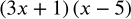.

Solution to #4. At x = 3, f(x) "touches" the x-axis, so the exponent of (x - 3) must be EVEN [2 ~ lowest degree] whereas at x = -3, f(x) "crosses" the x-axis, so the exponent of (x + 3) must be ODD [1 ~ lowest degree] BUT the "squiggle" at x = -3 indicates that the power MUST be 3 or greater...therefore 3 ~ lowest degree! The answer is choice #2 since the 1st one has a negative leading-term which isnt suggested by the graph...

Jay.
https://csetmathguru.weebly.com/

Last edited: Feb 2, 2019

Joined:
Jul 24, 2004
Messages:
486
2

Feb 2, 2019

Solution to #5. Since substituting h = 0 into the expression yields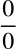, using L’Hopital’s Rule i.e. taking the derivative of every term in the numerator and denominator, we get: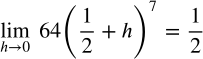.

Jay.
https://csetmathguru.weebly.com/

Joined:
Jul 24, 2004
Messages:
486
2

Feb 2, 2019

Solution to #6. Applying the Fundamental Theorem of Calculus: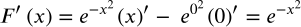.
Jay.
https://csetmathguru.weebly.com/

Joined:
Jul 24, 2004
Messages:
486
2

Feb 2, 2019

#8. SUBTEST 2. Suppose we have a random variable X where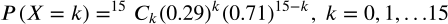. What is the standard deviation of X?

Jay.
https://csetmathguru.weebly.com/

Last edited: Feb 2, 2019

Joined:
Jul 24, 2004
Messages:
486
2

Feb 2, 2019

#9. SUBTEST 1. If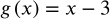,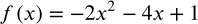, for what value(s)of x would the graph of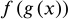intersect?

#10. Subtest 1. Solve: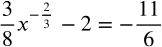Jay.
https://csetmathguru.weebly.com/

Last edited: Feb 18, 2019

Joined:
Jul 24, 2004
Messages:
486
2

Feb 2, 2019

Solution to #7. Since the volume of the soft-drink dispensed should exceed 12oz. only 1% of the time, we a mean such that: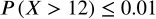.
Working the choices, with the NormalCdf command: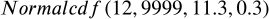, we get closest to the required probability of 0.01!

Jay.
https://csetmathguru.weebly.com/

Last edited: Feb 2, 2019

Joined:
Jul 24, 2004
Messages:
486
2

Feb 2, 2019

#11. SUBTEST 1. What is the y-intercept of the oblique asymptote of the function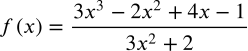?
Jay.
https://csetmathguru.weebly.com/

Joined:
Jul 24, 2004
Messages:
486
2

Feb 18, 2019

#12. SUBTEST 1. Find the inverse of the function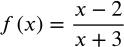and demonstrate that
it is the inverse function.

Jay.
https://csetmathguru.weebly.com/

Joined:
Jul 24, 2004
Messages:
486
2

Feb 18, 2019

#13. SUBTEST 2. Describe the transformation if the curve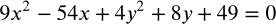has an image corresponding to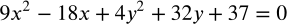.

Jay.
https://csetmathguru.weebly.com/

Joined:
Jul 24, 2004
Messages:
486
2

Feb 18, 2019

Solution to #8. Since X is a binomial random variable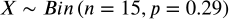, it's standard deviation is given by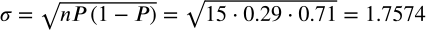.

Jay.
https://csetmathguru.weebly.com/

Joined:
Jul 24, 2004
Messages:
486
2

Feb 18, 2019

Solution to #9. Now,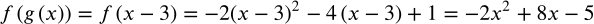.

So, solving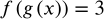, we get x = 2.
Solution to #10. Isolating the fractional power, we get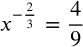.
Solving:.
Substituting into the original equation, we find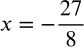.

Jay.
https://csetmathguru.weebly.com/

Last edited: Feb 18, 2019

Joined:
Jul 24, 2004
Messages:
486
2

Feb 18, 2019

Solution to #11. Via Long Division, we find the Oblique Asymptote to be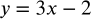, so the y-intercept would be -2Jay.
https://csetmathguru.weebly.com/

Joined:
Jul 24, 2004
Messages:
486